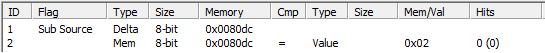Skip to content

# SubSource Flag

Note: most likely this technique is not needed for simple games. So, it's not mandatory for jr-devs.

The `SubSource` can be chosen in the Flag column of the Achievement Editor

Works similarly to `Add Source`, but the `Sub Source` flag makes the value in the memory address have a negative value.

Note 1: `Sub Source` is NOT a subtraction flag. It just makes the value be negative.

Note 2: The final line (without `Add Source` or `Sub Source`) is still added for the final comparison.

Using that `AddSource` usecase example, if we replaced it with the `Sub Source` and with the same values (`value(0x8010) = 1` and `value(0x8020) = 2`), the comparison would be `-1 + 2 > 0`, or `1 > 0`.

## Using SubSource to Count Specific Incrementslink

`SubSource` can also be used to track specific increases in addresses. The conditions below states that the current value in address `0x0080dc` less the value in the previous frame in the same address must be equal `2`. Effectively, this means that the condition is true whenever the value in address `0x0080dc` increases by exactly two.## Checking for a negative resultlink

If the result of your Sub Source operation is a negative number, it is possible to check for it. This is how negative numbers are represented:

• `-1` is `0xFFFFFFFF`
• `-2` is `0xFFFFFFFE`
• `-3` is `0xFFFFFFFD`
• `-4` is `0xFFFFFFFC`
• `-5` is `0xFFFFFFFB`
• `-6` is `0xFFFFFFFA`
• `-7` is `0xFFFFFFF9`
• and so on...

So, let's say you want to check if `value(0x8010) = 1` minus `value(0x8020) = 2` equals `-1`. This is how you would do it:This means `-2 + 1 = -1`, which is true.

Advanced: it is also possible to use straight negative numbers in the left section of your Sub/Add Source operations. To do so, remember to change the Type from `Mem` to `Value`, and use the values as explained above, such as `0xFFFFFFE5` for `-27`, and `0xFFFFFFE3` for `-29`, for example:This means `-2 + (-27) = -29`, which is `-2 - 27 = -29`, which is true.

Take care, though, if you use a straight negative number in a Sub Source flag, it will result in a double negative, turning the number back to positive. Take a look:This means `- (-2) + (-27) = -29`, which is `2 - 27 = -29`, which is `-25 = -29`, which is false.

## Changeloglink

Last 10 changes on this page:

• `[2020-01-15 08:23] meleu:` better example of a negative number
• `[2019-12-31 12:14] meleu:` cosmetic
• `[2019-02-17 23:42] meleu:` better example for "Using SubSource to Count Specific Increments"
• `[2019-02-17 21:05] meleu:` cosmetic
• `[2019-02-14 10:10] meleu:` fix broken link
• `[2019-02-12 13:25] meleu:` add heading to "Using SubSource to Count Increments"
• `[2019-02-12 13:18] meleu:` fix broken link
• `[2019-02-12 09:37] meleu:` Updated SubSource (markdown)